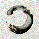809 views
809
With this, i calculate RSI of the HL2 of the volume and use like an oscillator, this will use to measure the strength of the trend and the "Volume Flow" to follow the trend.

I use like foundation the LazyBear "Volume Flow Indicator" "honor a quien honor merece"

Background:
I think the volume as the price could be represented by candles or other graphic to use indicators and strengthen their analysis, due to lack of registration of this it is first necessary to calculate a volume graph, if the candle traditionally negative price brand then the total volume is taken as negative for the period. An example of this is in the On Balance Volume indicator, the problem is that there is no way to analyze the volume using other methods. An approximate volume of the spread could be the use of the price spread to make a synthetic behavior

As traditionally is observed if Open> Close then the candle and the volume will be negative and vice versa; the next step, is estimate the amounts of the candle necessary to calculate the ratio to use for the volume and thus idealize their spread within the candle:

VLOW = Volume x Low
vHigh = x High Volume
VOpen = vClose
vClose = Volume x Close

This graph can show a stable synthetic form of fluctuations in the volume trend affected by price.
ideas, comments and suggestions (or corrections).They are always welcome
//
// Volume Flow Indicator Original by: [LazyBear]
// Oscillator and volume candle [devicemxl]
//

study(title = "Propagation Volumes and Trends", shorttitle="PVT")
length = input(130, title="VFI length")
coef = input(0.2)
vcoef = input(2.5, title="Max. vol. cutoff")
signalLength=input(14)
smoothVFI=input(false, type=bool)
ma(x,y) => smoothVFI ? sma(x,y) : x

typical=hl2
inter = log( typical ) - log( typical )
vinter = stdev(inter, 30 )
cutoff = coef * vinter * close
vave = sma( volume, length )
vmax = vave * vcoef
vc = iff(volume < vmax, volume, vmax) //min( volume, vmax )
mf = typical - typical
vcp = iff( mf > cutoff, vc, iff ( mf < -cutoff, -vc, 0 ) )

vfi = alma(sum( vcp , length )/vave, 3, 0, 6)
vfima=wma( vfi, signalLength )

plot( vfi, title="Volume Flow", color=red,linewidth=1)
plot( vfima, color=black,linewidth=2)

profundidad = input(20, title="RSI Length")
////////////
volumex=volume*(close>close ? 1 : -1)
V_OPEN  = V_CLOSE
V_CLOSE = close*volumex
V_HIGH  = max(max(high*volumex,V_CLOSE),V_OPEN)
V_LOW   = min(min(low*volumex,V_OPEN),V_CLOSE)
V_HL2   = ( V_HIGH + V_LOW ) / 2
///////////

side = open > close ? -1 : 1
vols = side * rsi(V_HL2,profundidad)

showHisto=input(true, type=bool)
plot(showHisto ? tendencia : na, color = (tendencia>tendencia ? (tendencia >0 ? green : red) : (tendencia >0 ? olive : maroon)), style=histogram, title="Power",linewidth=3)
//plot(showHisto ? tendencia : na, color = black, style=histogram, title="Tendencia", transp=85,linewidth=2)manoe
@manoe, Tnx Can i fit the "volume affected bar" in a "common bar"? I think Yes, but the idea is make a "synthetic" data model. We need the volume profile to show a "volume bar".

In i make a more refined idea of the model, with that you can put an other indicator like RSI, CCI, stoch, etc.

The idea behind the model is show a graphical affectation of the volume over the price, if you see the pics ( https://www.tradingview.com/x/TEswaAfA/) you can see how some times a great drop-down in price not is in volume and the model soft the effect of this, but other occasions the price not change too much but have a high volume, and the model reflect this.

Like another data modeling algorithm him function pretend simply show a simplification of the price affected by volume and reduce the "noise".

I tested at 1w and 1D a strategy RSI in OANDA:MXNUSD, AXP, LUK, JPM, QQQ, CX, etc. The strategy is a raw RSI without stop-loss or other thing, and the results are acceptable, the percent profitable is always over 50%, the profit factor sometimes ok too, but is a raw exercise:

//@version=2
strategy("Volume Spread Analysis3", shorttitle="VSA3")
//Analisis de propagacion en Volumen
// devicemxl --> TradingView Site

/// Values
volumex=log(volume)//*(close>close ? 1 : -1)
V_OPEN = n > 10 ? (V_CLOSE+V_OPEN)*0.5 : ((close*volumex)+(open*volumex))*0.5
V_CLOSE = n > 2 ? ( (close*volumex) + (high*volumex) + (low*volumex) + V_OPEN ) *0.25 : ( (close*volumex) + (high*volumex) + (low*volumex) ) / 3
//V_CLOSE = ( (close*volumex) + (high*volumex) + (low*volumex) + V_OPEN ) *0.25
///V_HIGH = max(max(high*volumex,V_CLOSE),V_OPEN)
//V_LOW = min(min(low*volumex,V_OPEN),V_CLOSE)
//V_HL2 = ( V_HIGH + V_LOW ) / 2
//senial=input(defval="CLOSE",title="Signal",type=string)
//ploter = ( senial == "CLOSE" ) ? V_CLOSE : ( senial == "OPEN" ) ? V_OPEN : ( senial == "HL2" ) ? V_HL2 : ( senial == "HIGH" ) ? V_HIGH : ( senial == "LOW" ) ? V_LOW : 0
//signal = plot(ploter, color=silver, style=circles)
//plotcandle(V_OPEN, V_HIGH, V_LOW, V_CLOSE, title='VSA', color = V_OPEN < V_CLOSE ? #f8f2ec : #53402d, wickcolor=black)

cciL = input(title="RSI Length", type=integer, defval=12, minval=3, maxval=210)
smas = input(title="signal", type=integer, defval=4, minval=3, maxval=210)
smok = input(title="smooth", type=integer, defval=6, minval=1, maxval=210)
b = true
a = 50
sourc = V_CLOSE//input(title="source",defval=close)

ccix = sma(rsi(sourc, cciL),smok)-a
cci14 = wma(ccix, smas)

plot(ccix, title="Main", color=navy, style=line, linewidth=1)
plot(cci14, title="Signal", color=red, style=line, linewidth=2)

//hline(50-a, title="Axis", color=gray, linestyle=dashed)
sc=input(10, title="Sobre-Compra" )
ei=input(20, title="Exuberancia Irracional" )
sv=input(-10, title="Sobre-Venta" )
pp=input(-20, title="Panico Psicotico" )
hline(sc)
hline(ei)
hline(sv)
hline(pp)
//fill(ei,pp, color=silver)
//fill(sc,sv, color=gray)

LG01 = ( ccix < pp )
LG02 = ( ccix > sv ) and ( ccix < sv )
SH01 = ( ccix > ei )
SH02 = ( ccix < sc ) and ( ccix > sc )
longCondition = LG01 or LG02
if (longCondition)
strategy.entry("My Long Entry Id", strategy.long, qty=1)

shortCondition = SH01 or SH02
if (shortCondition)
strategy.entry("My Short Entry Id", strategy.short, qty=0)